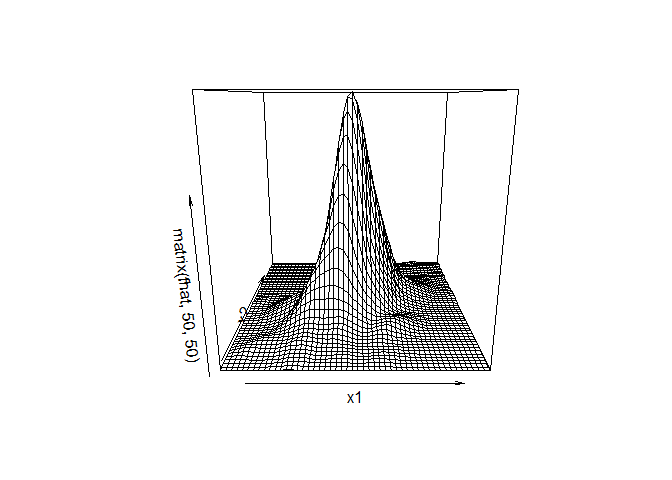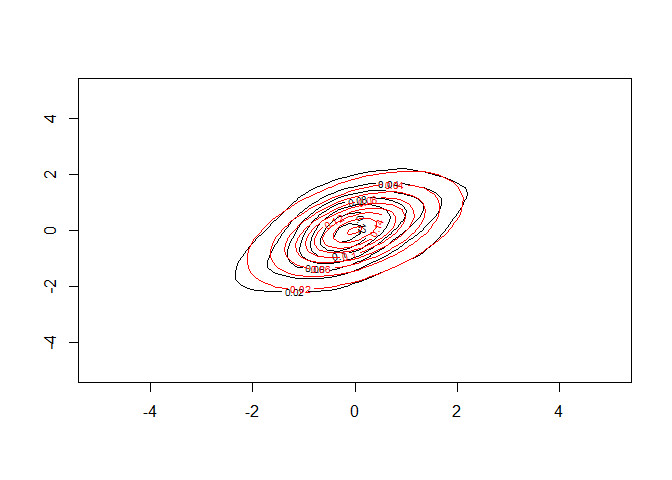# rlcv

The goal of rlcv is to provide a robust likelihood cross validation bandwidth selector for kernel density estimation. It allows for both uni-variate and multi-variate densities. It is resistant against fat-tailed distributions and outliers.

## Installation

You can install the development version of rlcv like so:

``install.packages('rlcv')``

## Example

This is a basic example which shows you how to use `rlcv` to fit a fat-tailed bivariate distribution:

``````library(rlcv)
## basic example code
# fat-tailed marginals + fat-tailed copula
library(copula)
ncop=tCopula(.5,df=5)
n=500
u=rCopula(n,ncop)
x1=qt(u[,1],5)
x2=qt(u[,2],5)
x=cbind(x1,x2)
fit=rlcv_d(x.obs=x)
# evaluation data
x1=x2=seq(-5,5,length=50)
x11=rep(x1,each=50)
x22=rep(x2,50)
fhat=kde_d(x.new=cbind(x11,x22),x.obs=x,,h=fit\$h)
f0=dCopula(cbind(pt(x11,5),pt(x22,5)),ncop)*dt(x11,5)*dt(x22,5)
# Mean squared errors
mean((f0-fhat)^2)
#>  2.131485e-05

persp(x1,x2,matrix(fhat,50,50))````````````contour(x1,x2,matrix(fhat,50,50))